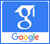# Asphalt layers## A qualitative method for determining the surfaces between asphalt layers using ABAQUS software

Published on: 25th April, 2019

OCLC Number/Unique Identifier: 8163588596

The analytical models are mainly combined with numerical equations for the problems of the pavement under the wheel load. Different assumptions can be considered, such as elastic asphalt and viscoelastic as well as static or dynamic load. Mainly on deformation at the bottom of asphalt and tension layers focus on subgrid. The pavement structure was considered as layers with uniform characteristics. Therefore, this analytical model calculates the three-dimensional contact tension between the wheel and the pavement and the shape of the contact area. Basis and subgrid are considered linear and the asphalt layers can be linear or viscoelastic. This model is based on the results of direct shear stress tests at an axial load constant. The curves obtained from this experiment can be defined by three parameters: the maximum shear stress (shear stress curve versus shear displacement), the interaction modulus between the layers (the same curve slope) and the friction coefficient after the failure. Due to the ability of ABACOUS software, this project is done with this software. One of the methods widely used to predict viscoelastic responses of asphalt mixtures is the finite element method. ABAQUS software is one of the tools that can simulate mixed asphalt behavior based on a finite element method, taking into account all the determinant parameters. The use of the Prony series is one of the common techniques for describing the viscoelastic behavior of asphalt mixtures in ABAQUS software. For this purpose, it is necessary to determine the parameters required for this field, including proven constants, moment elastic modulus, and asphalt mixture poison ratio. On the other hand, the determination of these parameters through testing in addition to spending time and costs requires laboratory equipment. Therefore, in this thesis, a three-dimensional finite element model with ABAQUS software was constructed to analyze the persistent pavement using theoretical relations without conducting the experiment. Also, viscoelastic behavior of common asphalt mixtures and time dependence of its responses at different temperatures can be modeled in ABAQUS software. After performing the shear stress test for different axial loads, different temperatures, with or without a single coil, they found that all parameters are temperature dependent and the coefficient of friction does not depend on the applied axial load. This new model improves the accuracy of the finite element model and its important role can be an analytic expression that includes all the variables that are effective in the problem.## Investigation and analysis of fracture failure and fatigue cracking in High-rise pavement using simulation software of ABAQUS

Published on: 27th May, 2019

OCLC Number/Unique Identifier: 8165128181

Assuming that the road infrastructure has been implemented in accordance with specifications and standards, poor adhesion between the two layers of asphalt mix can be a significant cause of pavement problems. The general problem observed with the weak adhesion between the layers is the slip failure. Slipping disruption in areas where transport acceleration increases, in areas where acceleration decreases or may occur in bumps. One of the criteria and a test method for measuring adhesion resistance between the hot mix asphalt layers is needed to improve the surface finish. The main objective of this study is to determine the effect of reducing the coefficient of friction between asphalt layers in the displacement of asphaltic layers. Because performing experimental experiments in the country is a deterrent to this goal, the use of analytical and numerical methods has been shown to play an important role in conducting studies. Therefore, in this paper, using vehicle simulation in ABAQUS software and analyzes, it has been found that decreasing the coefficient of friction (adhesion reduction) increases the interlayer deformation, which causes the surface of the pavement to fail. Three different thicknesses for asphalt cladding, including 4, 6, and 7 centimeters, and three different thicknesses for roller concrete layers of 18, 20 and 22 centimeters are used. Modeling and analysis of pavements with finite element method has been performed and the depth of the asphalt and tensile strain slope is calculated at the maximum level. The results show that the type of asphalt mix has a high impact on the amount of sloping and tensile strain at the maximum level. So that under different conditions it is estimated to be about 2-3 times in the amount of rotation at the surface. Also, the amount of groove and strain in the middle of the procedure is increased by a thickness of 11% the thickness of the roller concrete thickness has not changed, but the surface strain has been reduced by 9%.